# Fundamentals of Physics Study Set 2

Physics & Astronomy

## Quiz 12 : Equilibrium and ElasticityStudy FlashcardsLooking for Introductory Physics Homework Help?

## Quiz 12 :Equilibrium and Elasticity

Question TypeA 160-N child sits on a light swing and is pulled back and held with a horizontal force of 100 N.The magnitude of the tension force of each of the two supporting ropes is:
Free
Multiple Choice

B

Tags
Choose question tagFor a body to be equilibrium under the combined action of several forces:
Free
Multiple Choice

C

Tags
Choose question tagA massless meter stick on a horizontal frictionless table top is pivoted at the 80-cm mark.A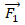force is applied perpendicularly to the end of the stick at 0 cm, as shown.A second force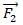(not shown)is applied perpendicularly to the stick at the 100-cm end of the stick.The forces are in the plane of the table top.If the stick does not move, the force exerted by the pivot on the stick: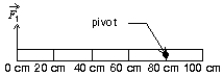Free
Multiple Choice

E

Tags
Choose question tagThe center of gravity coincides with the center of mass:
Multiple Choice
Tags
Choose question tagA 5.1-kg mass is located at the origin, and a 2.3-kg mass is located at x = 4.9 cm.Assuming g is constant, what is the location of the center of mass xcom, and the location of the center of gravity xcog, of the two masses?
Multiple Choice
Tags
Choose question tagWhich of the following is NOT in equilibrium?
Multiple Choice
Tags
Choose question tagWhich of the following is in static equilibrium?
Multiple Choice
Tags
Choose question tagThree identical uniform rods are each acted on by two or more forces, all perpendicular to the rods.Which of the rods could be in static equilibrium if an additional force is applied at the center of mass of the rod?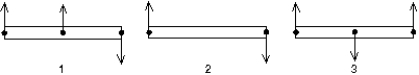Multiple Choice
Tags
Choose question tagThe location of which of the following points within an object might depend on the orientation of the object?
Multiple Choice
Tags
Choose question tagA net torque applied to a rigid object always tends to produce:
Multiple Choice
Tags
Choose question tagA massless meter stick on a horizontal frictionless table top is pivoted at the 80-cm mark.A force is applied perpendicularly to the end of the stick at 0 cm, as shown.A second force (not shown)is applied perpendicularly at the 60-cm mark.The forces are in the plane of the table top.If the stick does not move, the force exerted by the pivot on the stick: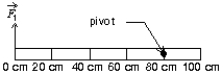Multiple Choice
Tags
Choose question tagFor a body to be equilibrium under the combined action of several forces:
Multiple Choice
Tags
Choose question tagTo determine if a rigid body is in equilibrium the vector sum of the gravitational forces acting on the particles of the body can be replaced by a single force acting at:
Multiple Choice
Tags
Choose question tagFor an object in equilibrium the sum of the torques acting on it vanishes only if each torque is calculated about:
Multiple Choice
Tags
Choose question tagA picture can be hung on a wall in three different ways, as shown.The tension in the string is: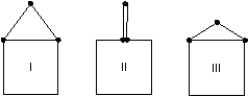Multiple Choice
Tags
Choose question tagA picture P of weight W is hung by two strings as shown.The magnitude of the tension force of each string is T.The total upward pull of the strings on the picture is: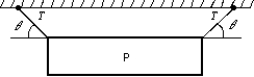Multiple Choice
Tags
Choose question tagA cube balanced with one edge in contact with a table top and with its center of gravity directly above the edge is in ________ equilibrium with respect to rotation about the edge and in ________ equilibrium with respect to rotation about a horizontal axis that is perpendicular to the edge.
Multiple Choice
Tags
Choose question tagThe conditions that the sum of forces and the sum of the torques both vanish:
Multiple Choice
Tags
Choose question tagA cylinder placed so it can roll on a horizontal table top, with its center of gravity below its geometrical center, is:
Multiple Choice